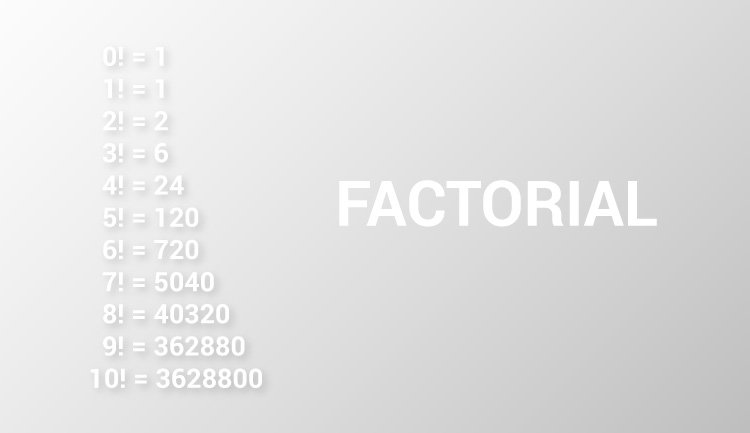# C Program to Find Factorial of a Number

In this example, you will learn to calculate the factorial of a number entered by the user.To understand this example, you should have the knowledge of the following C programming topics:

The factorial of a positive number n is given by:

``````factorial of n (n!) = 1 * 2 * 3 * 4....n
``````

The factorial of a negative number doesn't exist. And, the factorial of 0 is 1.` `

## Factorial of a Number

``````#include <stdio.h>
int main() {
int n, i;
unsigned long long fact = 1;
printf("Enter an integer: ");
scanf("%d", &n);

// shows error if the user enters a negative integer
if (n < 0)
printf("Error! Factorial of a negative number doesn't exist.");
else {
for (i = 1; i <= n; ++i) {
fact *= i;
}
printf("Factorial of %d = %llu", n, fact);
}

return 0;
}
``````

Output

```Enter an integer: 10
Factorial of 10 = 3628800
```

This program takes a positive integer from the user and computes the factorial using `for` loop.

Since the factorial of a number may be very large, the type of factorial variable is declared as `unsigned long long`.

If the user enters a negative number, the program displays a custom error message.

You can also find the factorial of a number using recursion.